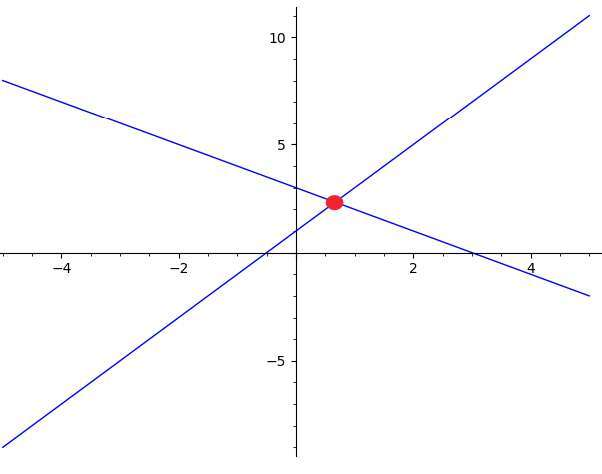# System of Equations: Graphing Method Calculator

Instructions: Use this calculator to solve a system of two linear equations using the graphical method. Please type two valid linear equations in the boxes provided below:Type a linear equation (Ex: y = 2x + 3, 3x - 2y = 3 + 2/3 x, etc.)Type another linear equation (Ex: y = 2x + 3, 3x - 2y = 3 + 2/3 x, etc.)(Optional) Minimum x =(Optional) Maximum x =

## More about the graphing method to solve linear systems

Systems of linear equations are very commonly found in different context of Algebra. The most commonly found systems in basic Algebra courses are 2 by 2 systems, which consist of two lines equations and two variables.

Such two-by-two systems often appear when solving word problems, proportion problems and assignment problems with constraint. Naturally, larger systems (with more variables and equations) also are common, here focus only on 2x2 systems, because those we can graph.### How to use graphing method

The graphing method consists of representing each of the linear equations as a line on a graph. Then, we need to find the intersection points between two lines, using the observation that the intersection point of the line (if it exists) will the solution of the system.

What happens if the intersection does not exist? That would be case if the lines are parallel without being the same line, in which case, there is no intersection. The rule is clear: when there is no intersection between the lines, there is no solution to the system.

There is a third case that can also happen: The lines could be parallel but actually identical (this is, they are the same line). So, how many intersection points do you have? Yes, your guess right: you have infinite intersection points, which means that you have infinite solutions.

### Solving Systems of equations by graphing answers

So, the methodology is simple: You start with a linear system, and the first thing you do is to graph the two linear equations.

Then, you look at the graph and assess whether the lines intersect at one point only (which happens if the lines have different slopes, in which case you have a unique solution.

If not, see if they parallel and different, in which case there are no solutions. Otherwise, if the two lines are equal, then we have infinite solutions.

### How do you solve a system of equations on a graphing calculator?

All systems have different ways of working. In this case of this graphing calculator, all you have to do is to type two linear equations, even if they are not completely simplified. The calculator first will try to get the lines into slope-intercept and will provide you with a graph and with an approximated estimate of the solution.

Different calculators will provide different outputs, but the great advantage of this calculator is that it will provide all the steps of the process.

### How do you write systems of equations from a graph?

Linear functions are univocally connected. This is, one linear equation is associated with one and one line only, and conversely, a line is associated with one linear equation and one linear equation only.

So, in order to write systems of equations from a graph, you need to work with each line separately. Take one line and identify two points on the line. With those two points you can compute the slope of the line.

Then, with the slope of the line and the y-intercept, you can write the equation of the line in slope-intercept form.